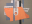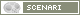OpenMusic DocumentationOM 6.6 User Manual > Visual Programming II > Abstraction > Recursive Patches
page précédentepage suivante

Recursive Patches

Recursion

In computer science, recursion is the application of a function within its own definition. This method allows to solve complex problems.

In order no to "call itself" infinitely, a recursive function must include a termination condition . A termination condition is a necessary condition so that the function doesn't call itself.

Examples

The "n!" Factorial Function

The "factorial" function calculates the product of all positive integers that are inferior or equal to a given n number. It is written "n!". It is a widespread example of recursive function. .

n! is defined as follows :

It can also be defined recursively :

For instance:

fact(3) = 1 x 2 x 3

fact(n) = 1 x 2 x 3 x ... x (n-1) x n

fact(n) = n x fact (n - 1)

For instance :

fact(3) = 3 x (fact(3-1))

fact(3) = 3 x (2 x fact(2-1))

fact(3) = 3 x (2 x 1)

And fact(1) = 1

The termination condition of the factorial function is fact(1) = 1.

It allows to calculate all the combinatorial possibilities of n elements, such as, for instance, the calculation of all possible melodic combinations of a group of notes.

The Fibonacci Suite

The Fibonacci suite calculates the sum of a number of integers inferior or equal to n. It is another type of recursive function, which is written "f!".

"f!" is defined as follows :

It can also be defined recursively :

fibo(n) = 1 + 2 + 3 + ... +(n-1) + n

For instance:

fact(6) = 1 + 2 + 3 + 4 + 5 + 6

fibo(n) = n + fibo (n-1)

For instance :

fibo(6) = 6 + (fibo (5))

page précédentepage suivante
A propos...(c) Ircam - Centre Pompidou